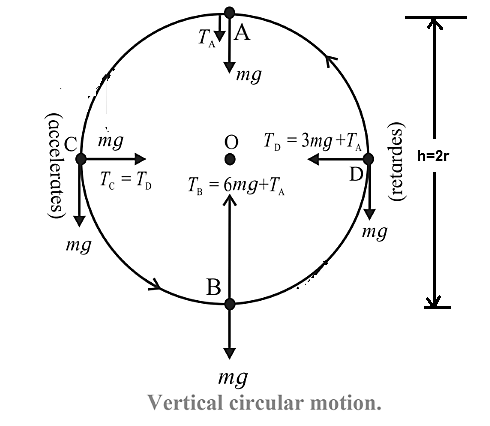# Using the energy conservation, derive the expressions for the minimum speeds at different locations along a

more_vert
Using the energy conservation, derive the expressions for the minimum speeds at different locations along a vertical circular motion controlled by gravity. Is zero speed possible at the uppermost point? Under what condition/s? Also prove that the difference between the extreme tensions (or normal forces) depends only upon the weight of the object

more_vert

verified

Consider a particle of mass m attached to a string and revolved in vertical circle of radius r, At every instant of motion  there are only two forces acting on the particle (a) its weight mg, vertically downwards, which is constant and

(b) the force due to the tension along the string, directed along the string and towards the centre.

Its magnitude changes periodically with time and location.The particle may not complete the circle if the string slackens before the particle reaches at the top. This requires that the particle must have some minimum speed.At the top position (Point A) : Let vbe the speed of the particle and Tthe tension in the string. Here both, weight mg and force due to tension TA are downwards, i.e. towards the centre. In this case, net force on the particle towards the center O is TA + mg is their resultant as the centripetal force.

∴ TA +mg = $$mvA^2\over r$$   ……..(1)

or minimum possible speed at this point (or if the motion is to be realized with minimum possible energy),

TA = 0

∴ 0 + mg =$$mvA^2\over r$$

$$\therefore{mv A^2\over r}$$= mg

That is the particle’s weight alone is the necessary centripetal force at the point A.

∴ vA= rg

∴ vA = $$\sqrt{rg} \ \ .......2$$

Discuss the necessary of radius of gyration define as on what factors does it depend and it does not depend